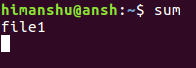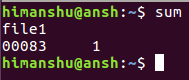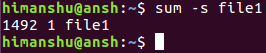# Linux sum Command Tutorial for Beginners (with Examples)

As you start spending more and more time working on the Linux command line, you tend to learn utilities that aren't very frequently used. Once such tool is sum, which only offers two features: display checksum and block count for input files. In this short tutorial, we will quickly discuss the basics of sum using some easy to understand examples.

But before we do that, it's worth mentioning that all examples in this tutorial have been tested on an Ubuntu 16.04 LTS machine.

## Linux sum command

As already mentioned above, the sum command gives you information about file checksum and the number of blocks a file is comprised of. Following is its syntax:

`sum [OPTION]... [FILE]...`

And here's how the tool's man page defines it:

`Print checksum and block counts for each FILE.`

Following are some Q&A-styled examples that should give you a good idea on how the tool works.

## Q1. How to use sum command?

Basic usage is fairly easy - just pass an input file to the command. For example:

`sum file1`

And here's the output the command produced on my system:

`54333     1`

The first entry is the checksum and the second one is the block count for the file

## Q2. How to make sum accept input from STDIN?

For this, all you have to do is to run the sum command without any input.

`sum`

As you press enter, the command will wait for you to provide input.Once the input file name is provided, just press ctrl+d, and you'll get the output.## Q3. How to change algorithm sum uses?

The tool offers two options: -r and -s. The former enables BSD sum algorithm, while the latter tells sum to use System V algo. Also, with -r, sum uses 1k block, while with -s, it uses 512 bytes blocks.

Here's an example:To know more about sum, you can use the standard --help and --version options. The former displays the complete help info, while the latter outputs the tool's version information.

## Conclusion

As you would have observed, the sum command is very easy to understand and use - the learning curve is very low. We've discussed almost all command line option the tool offers. To learn more about Sum, head to its man page.view as pdf |print